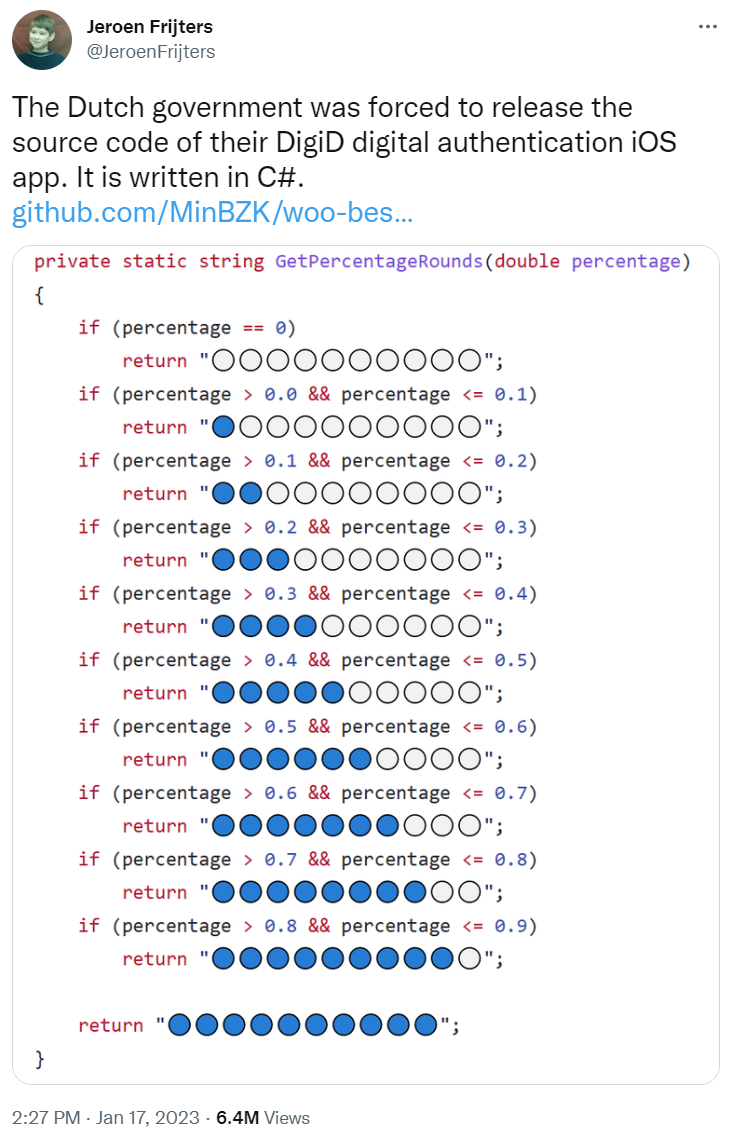# Fun with rating stars

Author: Paul King
Published: 2023-01-25 12:00AM

## Star Ratings

A recent tweet showed some C# code for producing a String of stars that might be used when displaying ratings on a website:Let’s have a look at several ways to do the same thing in Groovy.

``````def rating0(percentage) {
int stars = Math.ceil(percentage * 10)
}``````
``````def rating1(percentage) {
int stars = Math.ceil(percentage * 10)
"🔵" * stars + "⚪" * (10-stars)
}``````
``````def rating2(percentage) {
int skip = 10 - Math.ceil(percentage * 10)
"🔵🔵🔵🔵🔵🔵🔵🔵🔵🔵⚪⚪⚪⚪⚪⚪⚪⚪⚪⚪"[skip..<10+skip]
}``````
``````def rating3(percentage) {
switch(percentage) {
case 0             -> "⚪⚪⚪⚪⚪⚪⚪⚪⚪⚪"
case { it <= 0.1 } -> "🔵⚪⚪⚪⚪⚪⚪⚪⚪⚪"
case { it <= 0.2 } -> "🔵🔵⚪⚪⚪⚪⚪⚪⚪⚪"
case { it <= 0.3 } -> "🔵🔵🔵⚪⚪⚪⚪⚪⚪⚪"
case { it <= 0.4 } -> "🔵🔵🔵🔵⚪⚪⚪⚪⚪⚪"
case { it <= 0.5 } -> "🔵🔵🔵🔵🔵⚪⚪⚪⚪⚪"
case { it <= 0.6 } -> "🔵🔵🔵🔵🔵🔵⚪⚪⚪⚪"
case { it <= 0.7 } -> "🔵🔵🔵🔵🔵🔵🔵⚪⚪⚪"
case { it <= 0.8 } -> "🔵🔵🔵🔵🔵🔵🔵🔵⚪⚪"
case { it <= 0.9 } -> "🔵🔵🔵🔵🔵🔵🔵🔵🔵⚪"
default            -> "🔵🔵🔵🔵🔵🔵🔵🔵🔵🔵"
}
}``````

If you want you can test the various edge cases:

``````for (i in 0..3)
for (j in [0, 0.09, 0.1, 0.11, 0.9, 1])
println "rating\$i"(j)``````

## Increasing Robustness

The code examples here assume that the input is in the range `0 <= percentage <= 1`. There are several tweaks we could do to guard against inputs outside those ranges.

We could simply add an assert, e.g.:

``````def rating0b(percentage) {
assert percentage >= 0 && percentage <= 1
int stars = Math.ceil(percentage * 10)
}``````

Or, if we wanted to not fail, tweak some of our conditions, e.g. for `rating3`, instead of `case 0`, we could use `case { it ⇐ 0 }`.

We could push the checks into our types by making a special class, `Percent` say, which only permitted the allowed values:

``````final class Percent {
final Double value
Percent(Double value) {
assert value >= 0 && value <= 1
this.value = value
}
}``````

And we could optionally also use some metaprogramming to provide a custom `isCase` method:

``Double.metaClass.isCase = { Percent p -> delegate >= p.value }``

Which means we could tweak the rating method to be:

``````def rating3b(Percent p) {
switch(p) {
case 0.0d -> "⚪⚪⚪⚪⚪⚪⚪⚪⚪⚪"
case 0.1d -> "🔵⚪⚪⚪⚪⚪⚪⚪⚪⚪"
case 0.2d -> "🔵🔵⚪⚪⚪⚪⚪⚪⚪⚪"
case 0.3d -> "🔵🔵🔵⚪⚪⚪⚪⚪⚪⚪"
case 0.4d -> "🔵🔵🔵🔵⚪⚪⚪⚪⚪⚪"
case 0.5d -> "🔵🔵🔵🔵🔵⚪⚪⚪⚪⚪"
case 0.6d -> "🔵🔵🔵🔵🔵🔵⚪⚪⚪⚪"
case 0.7d -> "🔵🔵🔵🔵🔵🔵🔵⚪⚪⚪"
case 0.8d -> "🔵🔵🔵🔵🔵🔵🔵🔵⚪⚪"
case 0.9d -> "🔵🔵🔵🔵🔵🔵🔵🔵🔵⚪"
default   -> "🔵🔵🔵🔵🔵🔵🔵🔵🔵🔵"
}
}``````

We can be fancier here using `@EqualsAndHashCode` on the `Percent` class and/or using `@Delegate` on the `value` property, depending on how rich in functionality we wanted the `Percent` instances to be.

Alternatively, we could use a design-by-contract approach:

``````@Requires({ percentage >= 0 && percentage <= 1 })
def rating1b(percentage) {
int stars = Math.ceil(percentage * 10)
"🔵" * stars + "⚪" * (10-stars)
}``````